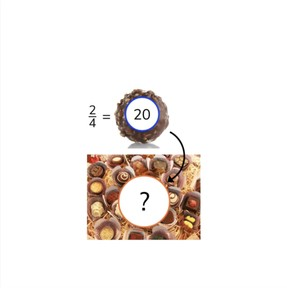Finding the whole given the part and the fraction

# Finding the whole given the part and the fraction

I can find the whole when I know the part and the fraction.8,000 schools use Gynzy92,000 teachers use Gynzy1,600,000 students use Gynzy

## General

Students learn to find the whole when they know the part and the fraction.

## Common core standard(s)

CCSS.MATH.CONTENT.3.NF.A.1

## Relevance

It is useful to learn how to do this, so you can use a fraction to determine the total.

## Introduction

Students must name the fraction represented by the fraction circle on the interactive whiteboard.

## Development

Remind students that a fraction is composed of a numerator and a denominator. Show a problem and tell students that 1//8 of the cars are red. To determine how many cars there are in total, you have to compose the whole fraction. Show that you multiply by 8 to get 8//8. Emphasize that the quantity of red cars (4) also must be multiplied by 8. There are 32 cars in total. Together with the students, go through these steps with the problems using the cake and strawberries. Use the next problems to check that students are able to determine the total by using a fraction when using a fraction bar. Finally explain fraction story problems to the students. Make sure that students follow the steps of solving a story problem, and that they select the correct numbers from the story problem.

Check that students are able to find the whole given the part and the fraction by asking the following question:
- Which steps do you take to determine the total when you have a fraction?

## Guided practice

Students first practice with multiple choice questions with visual support, then they must fill in the correct answers with visual support and finally students are given fraction story problems.

## Closing

Remind students of the importance of being able to find the total when given a fraction. To close the lesson, have students practice with two more problems given on the interactive whiteboard.

## Teaching tips

Students who have difficulty finding the total when given a fraction can start by practicing with simple fractions and by drawing or setting out manipulatives to help make the problem more visual.

## Instruction materials

Optional: manipulatives

### The online teaching platform for interactive whiteboards and displays in schools

• Save time building lessons

• Manage the classroom more efficiently

• Increase student engagement